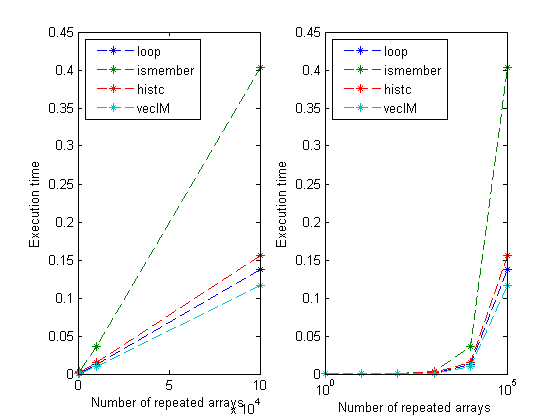Inverse Mapping (update)

In a follow up comment to the inverse mapping post, Lucio noted that loopFR could be fully vectorized and he is right. Rather than update that post directly, I thought I republish the loop-free code here and compare times again.

Contents

Tidy up to start.

clear
format short g

Sample Input and Results

Supppose array a contains values that we expect to see in another array, Minit.

a = [1 3 5 7 8]
Minit = [1 3 5; ...
3 5 7; ...
3 7 8]
a =
1     3     5     7     8
Minit =
1     3     5
3     5     7
3     7     8

Let's build a replacement matrix for Minit in which its values are replaced with their indices in the list a.

Mwanted = [1 2 3; ...
2 3 4; ...
2 4 5]
Mwanted =
1     2     3
2     3     4
2     4     5

Algorithms

We previously compared these algorithms:

• loop (via loopFR)
• ismember
• histc

Here's loopFR, for reference:

dbtype loopFR
1     function M1 = loopFR(M,A)
2     % loopFR replaces M with its indices from A.
3
4     % First set up the inverse mapping array.  Initialize it to zero, and the
5     % size is the maximum number the array.  Note that I get away with this
6     % here because all of my numbers in both A and M are positive integers.
7     imap = zeros(1,max(A));  % inverse mapping array
8     % Now fill the inverse map, but placing in the location specified in each
9     % element of A, the index of that element in A.
10    % If A = [1 5 4], then imap = [1 0 0 3 2].
11    imap(A) = 1:length(A);
12    M1 = M;
13    for k = 1:numel(M)
14        M1(k) = imap(M(k));
15    end

and let's now add the fully vectorized version, vecIM to our comparison.

type vecIM
function M1 = vecIM(M,a)
%vecIM Inverse mapping.
imap(a) = 1:length(a);
M1 = imap(M);

Time the New Algorithm and Retime the Former Ones

N = [1 10 100 1000 10000 100000];  % repetitions to test
t = zeros(length(N),4); % set up the timing collection array
for n = 1:length(N)
% Make a larger matrix to search.
% loopFR
M = repmat(Minit,N(n),1);
tic;
M1 = loopFR(M,a);
t(n,1) = toc;
% ismember
tic;
[M2,M2] = ismember(M,a);
t(n,2) = toc;
% histc
tic;
[M3,M3] = histc(M,a);
t(n,3) = toc;
% vecIM
tic;
M4 = vecIM(M,a);
t(n,4) = toc;
if ~isequal(M1,M2,M3,M4)
disp('oops')
end
end

Timing Results

disp('       Counter     loop       ismember     histc        vecIM')
disp([N' t]);
subplot(1,2,1)
plot(N', t, '--*')
xlabel('Number of repeated arrays')
ylabel('Execution time')
legend({'loop','ismember','histc' 'vecIM'},'Location', 'NorthWest')
subplot(1,2,2)
semilogx(N', t, '--*')
xlabel('Number of repeated arrays')
ylabel('Execution time')
legend({'loop','ismember','histc' 'vecIM'},'Location', 'NorthWest')
Counter     loop       ismember     histc        vecIM
1  9.0514e-005   0.00028691  2.2349e-005  5.5594e-005
10  7.2635e-005   0.00024612  3.1568e-005  5.9225e-005
100   0.00018522   0.00054839   0.00018131   0.00014471
1000     0.001263    0.0034977    0.0013865   0.00097079
10000     0.012539     0.035746     0.014809     0.010385
1e+005      0.13758      0.40348      0.15652      0.11654